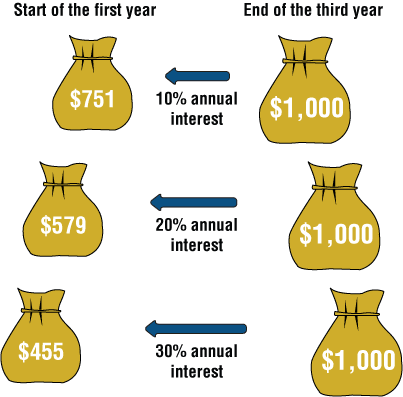# How Do Interest Rates Affect Present Value?

The higher the interest rate, the faster money grows. In the following example we’ll see that for different interest rates we should deposit different sums of money in order to reach \$1,000 in three years.

As shown by the following chart, when the annual interest rate is 10%, \$751 must be invested today. When the annual interest rate is 20%, it is sufficient to invest \$579 today, and when the annual interest rate soars to 30%, \$455 is sufficient.

Note the direction of the arrows in the chart: The sum we want to receive three years from now (\$1,000) is our target sum, which we have “moved backwards”. In order to obtain its present value according to each of the three interest rates:• When the annual interest rate is 10%, the present value of \$1,000 is \$751.

• When the annual interest rate is 20%, the present value of \$1,000 is \$579 (a decrease).

• When the annual interest rate is 30%, the present value of \$1,000 is \$455 (another decrease).

In conclusion:

As the interest rate increases, the present value decreases.

## Remembering Something Simple

The present value of any sum of money we receive now is the exact sum received. For example, if we received \$1,000 today, its present value  is exactly \$1,000.

If we receive the \$1,000 at any time in the future (a day, a month, or a year from now), its present value will be less than \$1,000 (the time that will elapse before we receive the money erodes its value, as shown in the illustration). Emphasizing this simple conclusion will help us to shorten the following explanations.